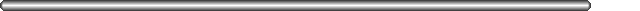# CLASS 21# Machine Instructions (continued)

## Format 2 Instructions

Format two instructions are the branch instructions, and the "sethi" instruction.

### Branch Instructions

The fields in a branch instruction look like this:
```Width:   2  1   4   3            22
--------------------------------------
|00| |    |   |                      |
--------------------------------------
Name:    op a cond op2       displacement

op:  always 00
a:   if 1, the branch is annulled
op2: specifies the type of test used (integer/floating point/
coprocessor) for integer tests -- the only kinds we'll use
-- this field is always 010
displacement:  the number of instructions between the current
instruction and the target of the branch.  This is a two's
complement number.
```
Note that the address of the branch is given as a distance (number of instructions) from the current instruction. This is called PC-relative addressing, since the PC is pointing at the current instruction which it is evaluated. So to calculate the new address to branch to, the processor sets:
```        PC = PC + displacement * 4
```
The reason we multiply by 4 is that each instruction is 4 bytes long, and memory addresses are given in terms of bytes. The effect of this is that if the displacement field of a branch contains the number 3, the branch target is 3 instructions away, NOT stored at location 3 in memory.

Note that the range of a branch is limited -- we can only branch to locations that are +/- about 8 MB from the current location at any point in time. However, this really isn't a problem, since branches are used almost exclusively to branch to locations inside the current subroutine. It is very rare that a subroutine is more than 16 MB long. This would mean that the subroutine had 4 million separate instructions, so it would have at least 4 million lines when written in assembly. That's a long subroutine.

### The sethi Instruction

We noted above that one can only include relatively small immediate values in instructions -- values in the range -4096 to +4095. How does one get a larger immediate value into a register? The answer is the "sethi" instruction.

The fields in a sethi instruction look like this:

```
Width:   2    5    3            22
-------------------------------------
|00|     |100|                      |
-------------------------------------
Name:    op   rd  op2          imm22

op:  always 00
rd:  the destination register
op2: always 100
displacement: the high 22 bits of the immediate value.

```
The sethi instruction is used to load 22 bits into the HIGH 22 bits of a register. The instruction looks like this:
```        sethi   22-bit-immediate, rd
```
Say you coded the following instruction:
```        sethi   0x12345, %l0
```
The instruction would be encoded as follows:
```         00 10000 100 0000010010001101000101
Name:    op   rd  op2          imm22
```
The result of executing this instruction would be that register %l0 would contain:
```         0000010010001101000101 0000000000
```
Note how the 22 bits have been moved to the high end of the register, and the low 10 bits have been filled with zeros.

How do you use this to load a 32 bit constant into a register? You need two instructions. First, load the high 22 bits using the sethi instruction. Then, use an "add" or an "or" instruction to get the low 10 bits in.

Say you want to load the 32 bit constant 0x30cf0034 into register %o0. First, you need to get the high 22 bits out of that number. One way is simply to ask the assembler to do it for you:

```        sethi %hi(0x30cf0034), %o0
```
For doing the bit shifting, and for obtaining the MSbits or the LSbits, there are two operators provided, which are defined as:
```%hi(x),  x >> 10
%lo(x),  x  & 0x3ff
```
The %hi() operator means right-shift the bit pattern by 10 places. This throws away the low 10 bits and puts the high 22 bits into position. Note that the assembler is doing the right-shift BEFORE it assembles the instruction -- you are just using it like a calculator here to do your work for you.

Next, you would load in the low 10 bits of the number into the register. You can do this with an "add" or an "or". The assembler recognizes the %lo() operator, which returns the low 10 bits of its argument. So you could next write:

```        add     %o0, %lo(0x30cf0034), %o0
```
and that would complete the process.

Finally, for the convenience of us humans, the assembler recognizes this command:

```        set     0x30cf0034, %o0
```
as a synonym for:
```        sethi %hi(0x30cf0034), %o0